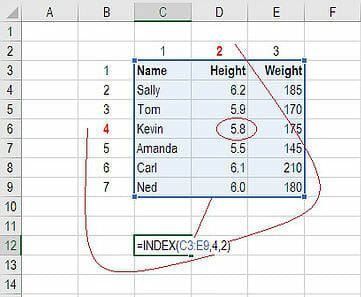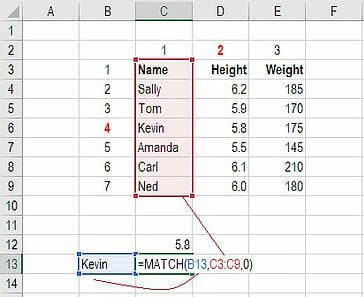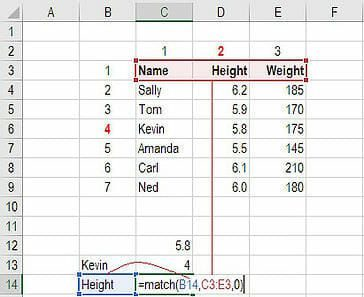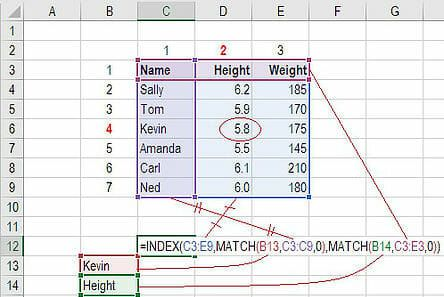# INDEX MATCH in Excel

The INDEX MATCH MATCH formula is the combination of two functions in Excel: INDEX and MATCH.

=INDEX() returns the value of a cell in a table based on the column and row number.

=MATCH() returns the position of a cell in a row or column.

Combined, the two formulas can look up and return the value of a cell in a table based on vertical and horizontal criteria.  For short, this is referred to as just the Index Match function.

### #1 How to use the INDEX formula

Below is a table showing people’s name, height and weight. We want to use the INDEX formula to look up Kevin’s height… here is an example of how to do it.

1. Type “=INDEX(” and select the area of the table then add a comma
2. Type the row number for Kevin, which is “4” and add a comma
3. Type the column number for Height, which is “2” and close the bracket
4. The result is “5.8”### #2 How to use the MATCH formula

Sticking with the same example as above, let’s use MATCH to figure out what row Kevin is in.

1. Type “=MATCH(” and link to the cell containing “Kevin”… the name we want to look up
2. Select the all the cells in the Name column (including the “Name” header)
3. Type zero “0” for an exact match
4. The result is that Kevin is in row “4”Use MATCH again to figure out what column Height is in.

1. Type “=MATCH(” and link to the cell containing “Height”… the criteria we want to look up
2. Select the all the cells across the top row of the table
3. Type zero “0” for an exact match
4. The result is that Height is in column “2”### #3 How to combine INDEX and MATCH

Now we can take the two MATCH formulas and use them to replace the “4” and the “2” in the original INDEX formula. The result is an INDEX MATCH MATCH formula.`https://corporatefinanceinstitute.com/resources/excel/study/index-match-formula-excel/`